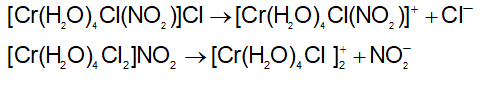Courses

# Coordination Compounds 15-12-2018

## 41 Questions MCQ Test AAKSC Chemistry Mock test Series | Coordination Compounds 15-12-2018

Description
This mock test of Coordination Compounds 15-12-2018 for Class 12 helps you for every Class 12 entrance exam. This contains 41 Multiple Choice Questions for Class 12 Coordination Compounds 15-12-2018 (mcq) to study with solutions a complete question bank. The solved questions answers in this Coordination Compounds 15-12-2018 quiz give you a good mix of easy questions and tough questions. Class 12 students definitely take this Coordination Compounds 15-12-2018 exercise for a better result in the exam. You can find other Coordination Compounds 15-12-2018 extra questions, long questions & short questions for Class 12 on EduRev as well by searching above.
QUESTION: 1

### The EAN of platinum in potassium hexachloroplatinate (IV) is:

Solution:

Effective atomic no. (EAN) = 78-4+2x6=86.

QUESTION: 2

### The hybridization of Fe in K4[Fe(CN)6] complex is:

Solution:

Electronic configuration of Fe2+ in K4Fe(CN)6 is: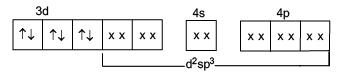x x Electron pair donated by CN

QUESTION: 3

### The colour of [Ti(H2O)6]3+ is due to:

Solution:

the d-d-excitation is responsible for colour of T i(H2O)3+6 which has one unpaired electron.

QUESTION: 4

[Cr(NH3)6]3+ ion is:

Solution:

[Cr(NH3)6]3+ has three unpaired electrons. Electronic configuration of Cr3+ in Cr(NH3)6 is: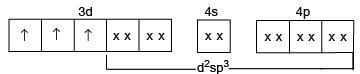QUESTION: 5

Which will give a white precipitate with AgNO3 in aqueous solution?

Solution: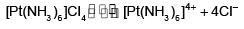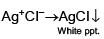QUESTION: 6

EDTA is a ........ligand.

Solution: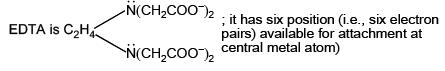QUESTION: 7

The number of ions formed on dissolving one molecule of FeSO4.(NH4)2SO4.6H2O in water is:

Solution:

It is a double salt: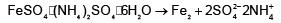QUESTION: 8

Aqua regia reacts with Pt to yield:

Solution:

It is a fact

QUESTION: 9

In [Cr(NH3)4CI2]CI the ligands are:

Solution:

Atoms or group donating electron pair to metal are ligands.

QUESTION: 10

Which possesses tetrahedral shape (sp3 - hybridization of central atom?

Solution:

All involves sp3 - hybridization.

QUESTION: 11

Which of the following is p complex?

Solution:

In π-complex, organic ligand use their π system to bond with metal, e.g., ferrocene.

QUESTION: 12

Which complex has square planar shape & dsp2- hybridization?

Solution:

All involve dsp2-hybrdization

QUESTION: 13

Formula of ferrocene is:

Solution:

Ferroc ene is cyclopentadienide iron complex.

QUESTION: 14

The number of precipitable halide ions in [Pt(NH3)CI2Br]CI is:

Solution: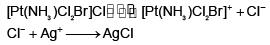QUESTION: 15

Which statement about coordination number of metal ion is true?

Solution:

The characteristics of coordination number.

QUESTION: 16

The correct name of the compound [Cu(NH3)4](NO3)2, according to IUPAC system is:

Solution:

Follow IUPAC rules.

QUESTION: 17

The formula of a carbonyl complex of (CO)n CO(CO)n, in  which there is a single covalent Co-Co bond is?

Solution:

Co shows zero oxidation state in its carbonyl  & follow EAN rule

QUESTION: 18

Of the following complexes, the one with the largest value of the crystal field splitting is:

Solution:

CN- ligand has strong ligand field so, value of CFSE is higher

QUESTION: 19

The IUPAC name of [CoCI(NO2)(en)2]CI is:

Solution:

Follow IUPAC rules.

QUESTION: 20

In which complex is the transition metal in zero oxidation state?

Solution:

Ni in Ni(CO)4 has zero oxidation stae.

QUESTION: 21

Which is low spin complex?

Solution:

Both CN- and NO2- are strong field ligands.

QUESTION: 22

From the stability constant (hypothetical values) given below, predict which is the strongest ligand?

Solution:

Higher is the stability constant of ligand, lesser is its dissoc iation, more is its stability.

QUESTION: 23

Which of the following complex species do not involve d2sp3-hybridization?

Solution:

[Cr(NH3 )6 ][Co(CN)6 ] is isomer to [Co(NH3 )6 ][Cr(CN)6 ] , i.e., ligands are changed in complex anion and complex cation.

QUESTION: 24

Which of the following compounds would exhibit coordination isomerism?

Solution:

S-atom is donor in SCN and N-atom is donor in NCS. The linkage isomerism arises when ligand has two possibilities to attach on central atom.

QUESTION: 25

[(C6H5)2Pd(SCN)2] and [(C6H5)2Pd(NCS)2] are:

Solution: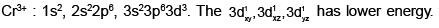QUESTION: 26

[Cr(H2O6]CI3 (at. no. of Cr=24) has a magnetic moment of 3.83 BM. The correct distribution of 3d-electrons in the chromium of the complex:

Solution:

Follow IUPAC rules.

QUESTION: 27

The hypothetical complex chloro diaquatriammine cobalt(III) chloride can be represented as:

Solution:

Chlorodiaquatriamminecobalt(Ill) chloride can be represented as [CoCl(NH3)3(H2O)2]Cl2.

QUESTION: 28

In the silver plating of Cu, K[Ag(CN)2] is used instead of AgNO3. The reason is:

Solution:

Copper being more electropositive readily precipitate silver from their salt (Ag+) solution.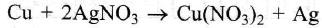In K[Ag(CN)2] solution a complex anion [Ag(CN)2] is formed so Ag+ ions are less available in the solution and Cu cannot displace Ag from this complex ion.

QUESTION: 29

The complex used as an anticancer agent is:

Solution:

d6-cation with low spin ha selectronic congiguration t62ge0g

Total energy= (-0.4D0per e- x6)
(-0.4D0 per e- x6)

+(e- paring energy of 3 pairs)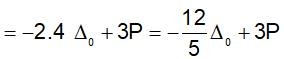QUESTION: 30

Low spin complex of d6-cation in an octahedral field will have the following energy:

Solution: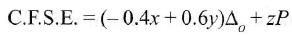where x = number of electrons occupying t2g orbital

y = number of electrons occupying eorbital

z = number of pairs of electrons

For low spin d6 complex electronic configuration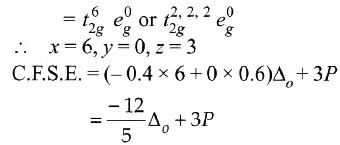QUESTION: 31

An excess of AgNO3 is added to 100 mL of a 0.01 M solution of dichlorotetraaquachromium (III) chloride. The number of moles of AgCI precipitated would be:

Solution:

Prussian blue is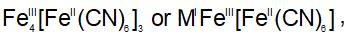where MI is Na,K, Rb, Li, Cs.

QUESTION: 32

The probable formula for prussian blue is:

Solution:
QUESTION: 33

Among the following identify the species with an atom of +6 oxidation state:

Solution:
QUESTION: 34

The type of isomerism, present in nitropentaamminechromium(III) chloride is:

Solution:

A square planar complex results from dsp2-hybridisation involving (n-1)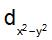, ns,np,npx and npy, atomic orbitals.

QUESTION: 35

In the complexes [Fe(H2O)6]3+, [FeCl6]3-, [Fe(C2O4)3]3- and [FeCI6]3- more stability is shown by:

Solution:

The central metal ion is Fe3+ and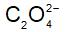is negative bi-dentate ligand which forms more stable complex than neutral or mono-dentate ligand.

QUESTION: 36

Among [Ni(CO)4],[Ni(CN)4]2- and [NiCI4]2- species the hybridisation states of Ni atom are respectively:

Solution:

The electronic configuration of Ni in [Ni(CN)4]2-, :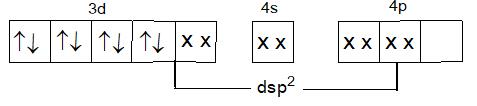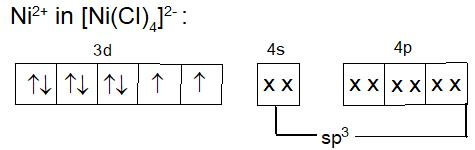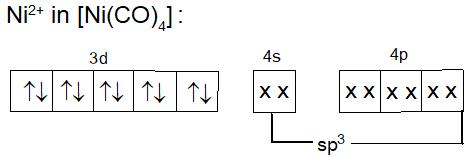QUESTION: 37

Ammonia forms the complex [Cu(NH3)4]2+ with copper ions on alkaline solution but not in acid solution. The reason for it is:

Solution:

It is a reason for given fact.

QUESTION: 38

The correct order of magnetic moments (only spin value in BM) among it:

Solution:

Mn2+, Fe2+ and Co2+ have 3d5 , 3d6, and 3d7 configuration respectively having sp3, d2dp3, dsp2 leaving 5,0 and 1 unpaired electrons. Pairing occurs in Fe2+ & Co2+ .

QUESTION: 39

The IUPAC name of [Ni(NH3)4][NiCI4] is:

Solution:

Follow IUPAC rules.

QUESTION: 40

A solution containing 2.675g of CoCI3.6NH3 (molar mass=267.5 g mol-1) is passed through a cation exchanger. Thechloride ions obtained in solution were treated with excess of AgNO3 to give 4.78 g of AgCI (molar mass = 143.5 g mol-1). The formula of the complex is:

(At. mass of Ag =108 u)

Solution:

Meq. of Cl- CIoutside the sphere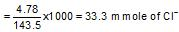= 0.033 mole of CI-

∴ WCl = 1.1825g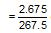or 0.01 mole of complex gives 0.01 x n mole of Cl-

∴ 0.01 x n = 0.033 ∴ n = 3
Thus, formula is [Co(NH3)6]CI3

QUESTION: 41

The ionization isomer of (Cr(H2O)4CI(NO2)]CI is:

Solution: